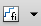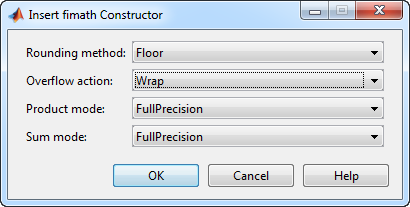## fimath Object Construction

### fimath Object Syntaxes

The arithmetic attributes of a `fi` object are defined by a local `fimath` object, which is attached to that `fi` object. If a `fi` object has no local `fimath`, the following default fimath values are used:

``` RoundingMethod: Nearest OverflowAction: Wrap ProductMode: FullPrecision SumMode: FullPrecision ```

You can create `fimath` objects in Fixed-Point Designer™ software in one of two ways:

• You can use the `fimath` constructor function to create new `fimath` objects.

• You can use the `fimath` constructor function to copy an existing `fimath` object.

To get started, type

```F = fimath ```

to create a `fimath` object.

```F = RoundingMethod: Nearest OverflowAction: Wrap ProductMode: FullPrecision SumMode: FullPrecision ```

To copy a `fimath` object, simply use assignment as in the following example:

```F = fimath; G = F; isequal(F,G)```
```ans = 1```

The syntax

```F = fimath(...'PropertyName',PropertyValue...) ```

allows you to set properties for a `fimath` object at object creation with property name/property value pairs. Refer to Setting fimath Properties at Object Creation.

### Building fimath Object Constructors in a GUI

When you are working with files in MATLAB®, you can build your `fimath` object constructors using the Insert fimath Constructor dialog box. After specifying the properties of the `fimath` object in the dialog box, you can insert the prepopulated `fimath` object constructor at a specific location in your file.

For example, to create a `fimath` object that uses convergent rounding and wraps on overflow, perform the following steps:

1. On the Home tab, in the File section, click New > Script to open the MATLAB Editor

2. On the Editor tab, in the Edit section, clickin the Insert button group. Click the Insert fimath... to open the Insert fimath Constructor dialog box.

3. Use the edit boxes and drop-down menus to specify the following properties of the `fimath` object:

• Rounding method = `Floor`

• Overflow action = `Wrap`

• Product mode = `FullPrecision`

• Sum mode = `FullPrecision`4. To insert the `fimath` object constructor in your file, place your cursor at the desired location in the file. Then click on the Insert fimath Constructor dialog box. Clicking closes the Insert fimath Constructor dialog box and automatically populates the `fimath` object constructor in your file:## SupportGet trial now